# Factorial_Designs (1).pptx

2. Jun 2023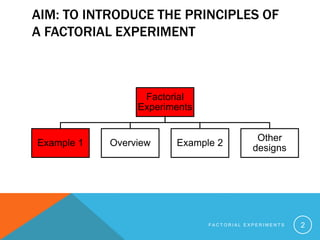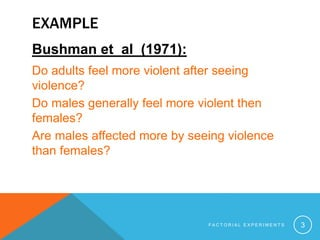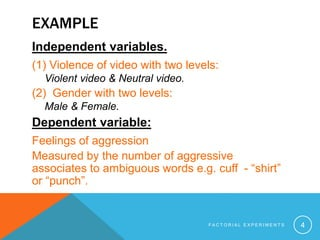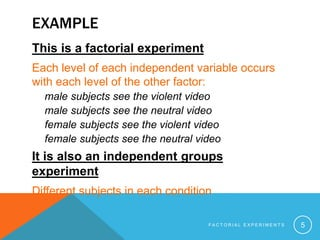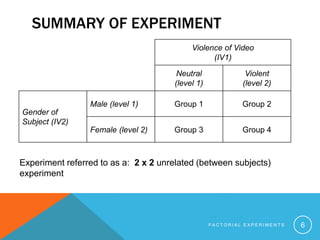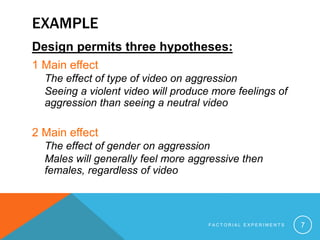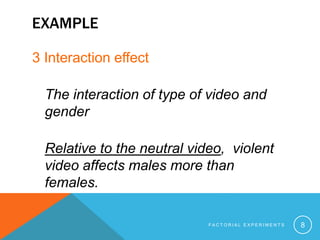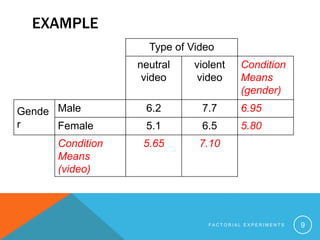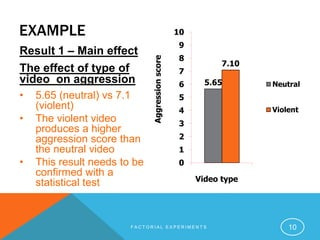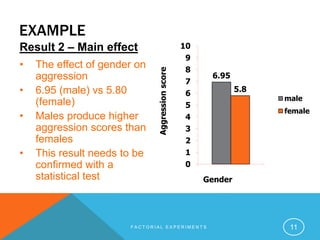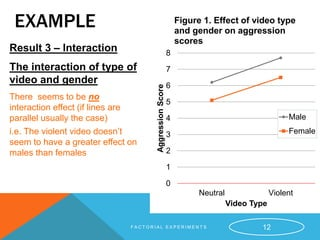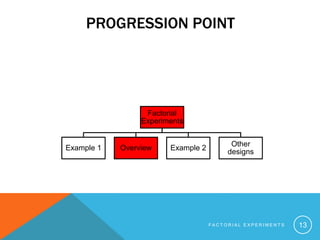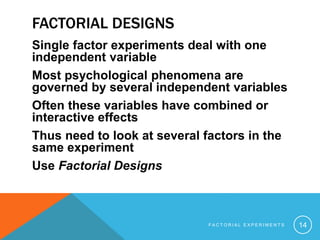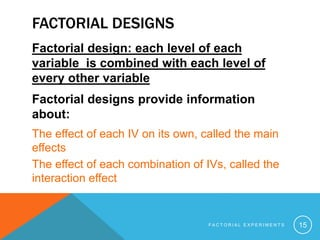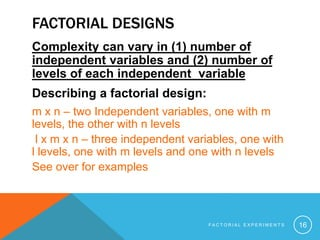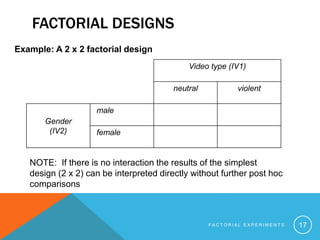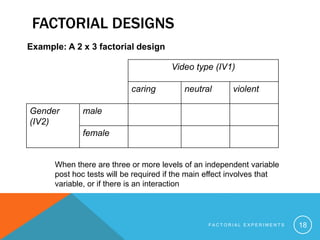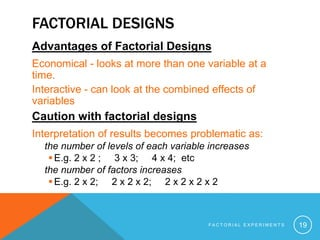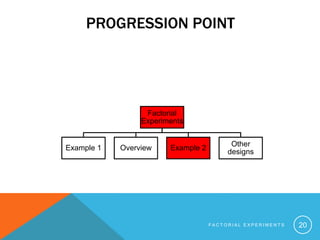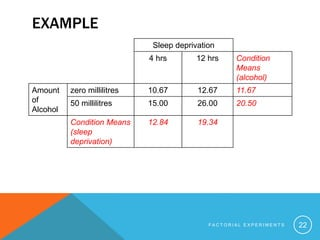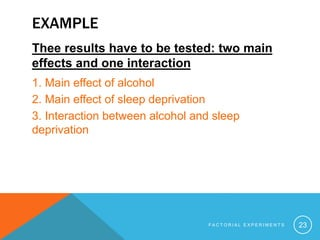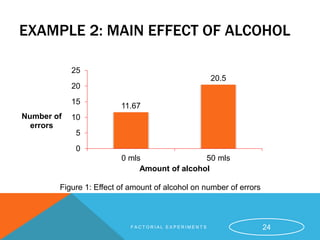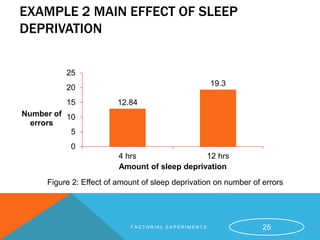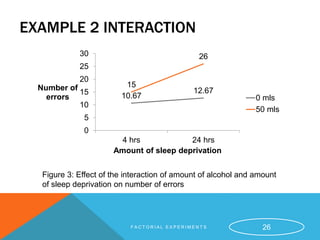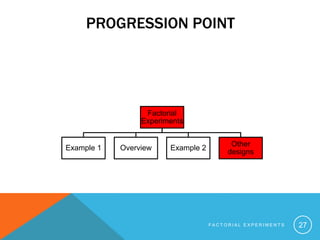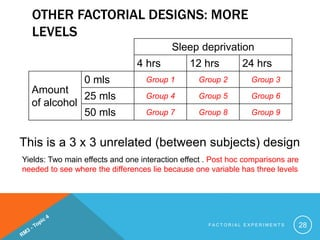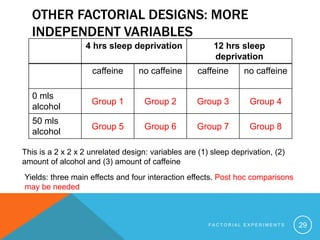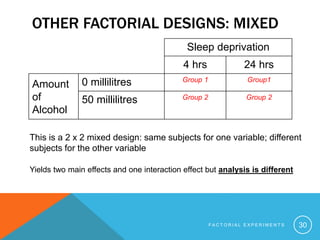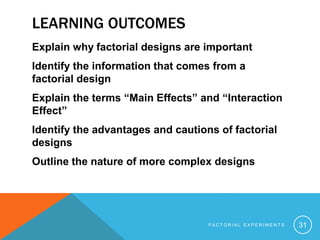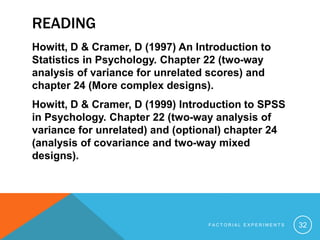1 von 32

### Factorial_Designs (1).pptx

• 2. AIM: TO INTRODUCE THE PRINCIPLES OF A FACTORIAL EXPERIMENT F A C T O R I A L E X P E R I M E N T S 2 Factorial Experiments Example 1 Overview Example 2 Other designs
• 3. EXAMPLE Bushman et al (1971): Do adults feel more violent after seeing violence? Do males generally feel more violent then females? Are males affected more by seeing violence than females? F A C T O R I A L E X P E R I M E N T S 3
• 4. EXAMPLE Independent variables. (1) Violence of video with two levels: Violent video & Neutral video. (2) Gender with two levels: Male & Female. Dependent variable: Feelings of aggression Measured by the number of aggressive associates to ambiguous words e.g. cuff - “shirt” or “punch”. F A C T O R I A L E X P E R I M E N T S 4
• 5. EXAMPLE This is a factorial experiment Each level of each independent variable occurs with each level of the other factor: male subjects see the violent video male subjects see the neutral video female subjects see the violent video female subjects see the neutral video It is also an independent groups experiment Different subjects in each condition F A C T O R I A L E X P E R I M E N T S 5
• 6. SUMMARY OF EXPERIMENT Violence of Video (IV1) Neutral (level 1) Violent (level 2) Gender of Subject (IV2) Male (level 1) Group 1 Group 2 Female (level 2) Group 3 Group 4 F A C T O R I A L E X P E R I M E N T S 6 Experiment referred to as a: 2 x 2 unrelated (between subjects) experiment
• 7. EXAMPLE Design permits three hypotheses: 1 Main effect The effect of type of video on aggression Seeing a violent video will produce more feelings of aggression than seeing a neutral video 2 Main effect The effect of gender on aggression Males will generally feel more aggressive then females, regardless of video F A C T O R I A L E X P E R I M E N T S 7
• 8. EXAMPLE 3 Interaction effect The interaction of type of video and gender Relative to the neutral video, violent video affects males more than females. F A C T O R I A L E X P E R I M E N T S 8
• 9. EXAMPLE Type of Video neutral video violent video Condition Means (gender) Gende r Male 6.2 7.7 6.95 Female 5.1 6.5 5.80 Condition Means (video) 5.65 7.10 F A C T O R I A L E X P E R I M E N T S 9
• 10. EXAMPLE Result 1 – Main effect The effect of type of video on aggression • 5.65 (neutral) vs 7.1 (violent) • The violent video produces a higher aggression score than the neutral video • This result needs to be confirmed with a statistical test 5.65 7.10 0 1 2 3 4 5 6 7 8 9 10 Aggression score Video type Neutral Violent F A C T O R I A L E X P E R I M E N T S 10
• 11. EXAMPLE Result 2 – Main effect • The effect of gender on aggression • 6.95 (male) vs 5.80 (female) • Males produce higher aggression scores than females • This result needs to be confirmed with a statistical test 6.95 5.8 0 1 2 3 4 5 6 7 8 9 10 Aggression score Gender male female F A C T O R I A L E X P E R I M E N T S 11
• 12. EXAMPLE Result 3 – Interaction The interaction of type of video and gender There seems to be no interaction effect (if lines are parallel usually the case) i.e. The violent video doesn’t seem to have a greater effect on males than females 0 1 2 3 4 5 6 7 8 Neutral Violent Aggression Score Video Type Figure 1. Effect of video type and gender on aggression scores Male Female F A C T O R I A L E X P E R I M E N T S 12
• 13. PROGRESSION POINT F A C T O R I A L E X P E R I M E N T S 13 Factorial Experiments Example 1 Overview Example 2 Other designs
• 14. FACTORIAL DESIGNS Single factor experiments deal with one independent variable Most psychological phenomena are governed by several independent variables Often these variables have combined or interactive effects Thus need to look at several factors in the same experiment Use Factorial Designs F A C T O R I A L E X P E R I M E N T S 14
• 15. FACTORIAL DESIGNS Factorial design: each level of each variable is combined with each level of every other variable Factorial designs provide information about: The effect of each IV on its own, called the main effects The effect of each combination of IVs, called the interaction effect F A C T O R I A L E X P E R I M E N T S 15
• 16. FACTORIAL DESIGNS Complexity can vary in (1) number of independent variables and (2) number of levels of each independent variable Describing a factorial design: m x n – two Independent variables, one with m levels, the other with n levels l x m x n – three independent variables, one with l levels, one with m levels and one with n levels See over for examples F A C T O R I A L E X P E R I M E N T S 16
• 17. FACTORIAL DESIGNS Video type (IV1) neutral violent Gender (IV2) male female F A C T O R I A L E X P E R I M E N T S 17 NOTE: If there is no interaction the results of the simplest design (2 x 2) can be interpreted directly without further post hoc comparisons Example: A 2 x 2 factorial design
• 18. FACTORIAL DESIGNS F A C T O R I A L E X P E R I M E N T S 18 Video type (IV1) caring neutral violent Gender (IV2) male female Example: A 2 x 3 factorial design When there are three or more levels of an independent variable post hoc tests will be required if the main effect involves that variable, or if there is an interaction
• 19. FACTORIAL DESIGNS Advantages of Factorial Designs Economical - looks at more than one variable at a time. Interactive - can look at the combined effects of variables Caution with factorial designs Interpretation of results becomes problematic as: the number of levels of each variable increases E.g. 2 x 2 ; 3 x 3; 4 x 4; etc the number of factors increases E.g. 2 x 2; 2 x 2 x 2; 2 x 2 x 2 x 2 F A C T O R I A L E X P E R I M E N T S 19
• 20. PROGRESSION POINT F A C T O R I A L E X P E R I M E N T S 20 Factorial Experiments Example 1 Overview Example 2 Other designs
• 21. EXAMPLE 2 (WITH SIGNIFICANT INTERACTION) Hypothetical experiment: Effects of alcohol and sleep deprivation on driving performance Independent variables 1. Amount of alcohol: 0 mls. vs 50 mls. 2. Amount of sleep deprivation: 4 hrs vs 12 hrs Dependent variable Number of mistakes on simulator F A C T O R I A L E X P E R I M E N T S 21
• 22. EXAMPLE Sleep deprivation 4 hrs 12 hrs Condition Means (alcohol) Amount of Alcohol zero millilitres 10.67 12.67 11.67 50 millilitres 15.00 26.00 20.50 Condition Means (sleep deprivation) 12.84 19.34 F A C T O R I A L E X P E R I M E N T S 22
• 23. EXAMPLE Thee results have to be tested: two main effects and one interaction 1. Main effect of alcohol 2. Main effect of sleep deprivation 3. Interaction between alcohol and sleep deprivation F A C T O R I A L E X P E R I M E N T S 23
• 24. EXAMPLE 2: MAIN EFFECT OF ALCOHOL 11.67 20.5 0 5 10 15 20 25 0 mls 50 mls Number of errors Amount of alcohol F A C T O R I A L E X P E R I M E N T S 24 Figure 1: Effect of amount of alcohol on number of errors
• 25. EXAMPLE 2 MAIN EFFECT OF SLEEP DEPRIVATION 12.84 19.3 0 5 10 15 20 25 4 hrs 12 hrs Number of errors Amount of sleep deprivation F A C T O R I A L E X P E R I M E N T S 25 Figure 2: Effect of amount of sleep deprivation on number of errors
• 26. EXAMPLE 2 INTERACTION 10.67 12.67 15 26 0 5 10 15 20 25 30 4 hrs 24 hrs Number of errors Amount of sleep deprivation 0 mls 50 mls F A C T O R I A L E X P E R I M E N T S 26 Figure 3: Effect of the interaction of amount of alcohol and amount of sleep deprivation on number of errors
• 27. PROGRESSION POINT F A C T O R I A L E X P E R I M E N T S 27 Factorial Experiments Example 1 Overview Example 2 Other designs
• 28. OTHER FACTORIAL DESIGNS: MORE LEVELS Sleep deprivation 4 hrs 12 hrs 24 hrs Amount of alcohol 0 mls Group 1 Group 2 Group 3 25 mls Group 4 Group 5 Group 6 50 mls Group 7 Group 8 Group 9 F A C T O R I A L E X P E R I M E N T S 28 This is a 3 x 3 unrelated (between subjects) design Yields: Two main effects and one interaction effect . Post hoc comparisons are needed to see where the differences lie because one variable has three levels
• 29. OTHER FACTORIAL DESIGNS: MORE INDEPENDENT VARIABLES 4 hrs sleep deprivation 12 hrs sleep deprivation caffeine no caffeine caffeine no caffeine 0 mls alcohol Group 1 Group 2 Group 3 Group 4 50 mls alcohol Group 5 Group 6 Group 7 Group 8 F A C T O R I A L E X P E R I M E N T S 29 This is a 2 x 2 x 2 unrelated design: variables are (1) sleep deprivation, (2) amount of alcohol and (3) amount of caffeine Yields: three main effects and four interaction effects. Post hoc comparisons may be needed
• 30. OTHER FACTORIAL DESIGNS: MIXED Sleep deprivation 4 hrs 24 hrs Amount of Alcohol 0 millilitres Group 1 Group1 50 millilitres Group 2 Group 2 F A C T O R I A L E X P E R I M E N T S 30 This is a 2 x 2 mixed design: same subjects for one variable; different subjects for the other variable Yields two main effects and one interaction effect but analysis is different
• 31. LEARNING OUTCOMES Explain why factorial designs are important Identify the information that comes from a factorial design Explain the terms “Main Effects” and “Interaction Effect” Identify the advantages and cautions of factorial designs Outline the nature of more complex designs F A C T O R I A L E X P E R I M E N T S 31
• 32. READING Howitt, D & Cramer, D (1997) An Introduction to Statistics in Psychology. Chapter 22 (two-way analysis of variance for unrelated scores) and chapter 24 (More complex designs). Howitt, D & Cramer, D (1999) Introduction to SPSS in Psychology. Chapter 22 (two-way analysis of variance for unrelated) and (optional) chapter 24 (analysis of covariance and two-way mixed designs). F A C T O R I A L E X P E R I M E N T S 32